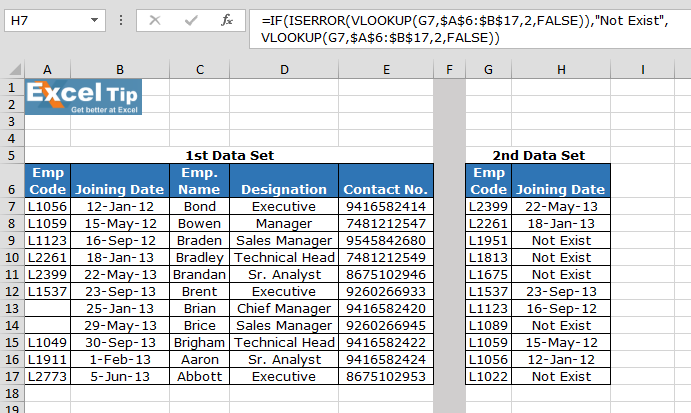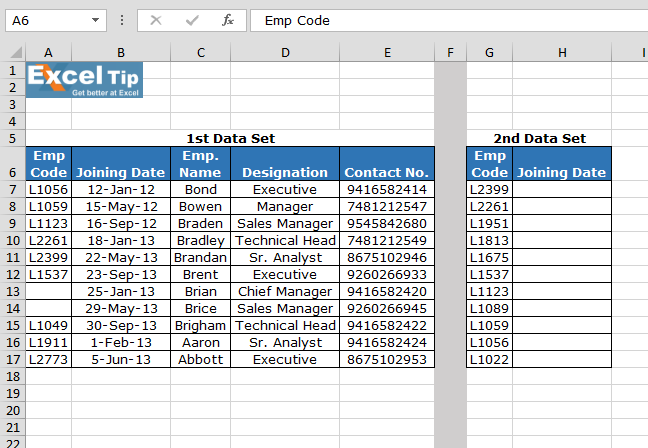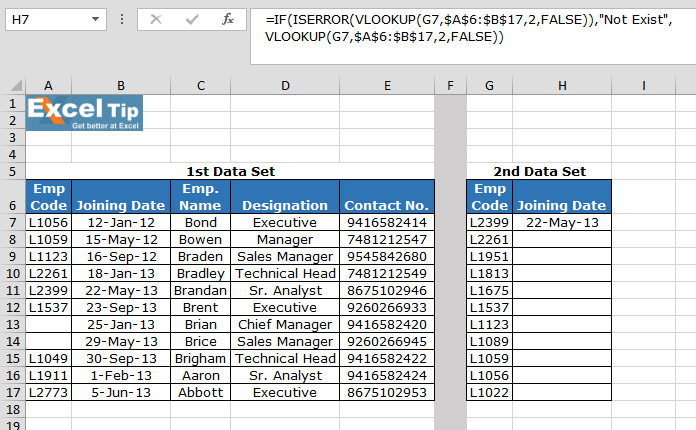# How to use the ISERROR function in ExcelIn this article, we will learn How to use the ISERROR function in Excel.

What is the ISERROR function ?

Whenever we operate formulas in Excel. Sometimes we get error values in response in Excel. But we don't want to see different error values. Instead, we will return output we want like value not found or value not existed. So excel assist us with a function which do not any return error values when used. This function over rules all error values like #N/A, #VALUE!, #REF!, #DIV/0!, #NUM!, #NAME?, or #NULL!.

ISERROR Function in Excel

ISERROR function checks for various errors in the condition like #N/A, #VALUE!, #REF!, #DIV/0!, #NUM!, #NAME?, or #NULL!.

Syntax:

 =ISERROR( formula or operation )

Formula: perform the operation inside the parentheses

Example :

All of these might be confusing to understand. Let's understand how to use the function using an example. Here we have two tables named 1st Data set and 2nd Dat set.Write the VLOOKUP formula in H7 cell.

 =IF(ISERROR(VLOOKUP(G3,\$A\$2:\$B\$13,2,FALSE)),"Not Exist", VLOOKUP(G3,\$A\$2:\$B\$13,2,FALSE))

Explanation:

Vlookup function checks the condition for the 2nd column of the 1st Data set matching EMP CODE in 1st Data set.

ISERROR function looks for any error and passes it on to the IF function.

IF function checks if an error occurs, it print “Not Exist” instead of the error.Copy the formula in other cells, select the cells taking the first cell where the formula is already applied, use shortcut key Ctrl + D.As you can see in the above image that formula is working fine. And the user can work without getting irritated by any error. If ISERROR function is not used, vlookup returning n/a error is the output.

Hope this article about How to use the ISERROR function in Excel is explanatory. Find more articles on IF condition formulas here. If you liked our blogs, share it with your fristarts on Facebook. And also you can follow us on Twitter and Facebook. We would love to hear from you, do let us know how we can improve, complement or innovate our work and make it better for you. Write to us at info@exceltip.com.

Related Articles :

IF with AND function : Implementation of logic IF function with AND function to extract results having criteria in Excel.

IF with OR function : Implementation of logic IF function with OR function to extract results having criteria in excel data.

How to use nested IF function : nested IF function operates on data having multiple criteria. The use of repeated IF function is nested IF excel formula.

SUMIFS using AND-OR logic : Get the sum of numbers having multiple criteria applied using logic AND-OR excel function.

Minimum value using IF function : Get the minimum value using the excel IF function and MIN function on array data.

How to use wildcards in excel : Count cells matching phrases using the wildcards in excel

Popular Articles :

How to use the IF Function in Excel : The IF statement in Excel checks the condition and returns a specific value if the condition is TRUE or returns another specific value if FALSE.

How to use the VLOOKUP Function in Excel : This is one of the most used and popular functions of excel that is used to lookup value from different ranges and sheets.

How to Use SUMIF Function in Excel : This is another dashboard essential function. This helps you sum up values on specific conditions.

How to use the COUNTIF Function in Excel : Count values with conditions using this amazing function. You don't need to filter your data to count specific values. Countif function is essential to prepare your dashboard.

Terms and Conditions of use

The applications/code on this site are distributed as is and without warranties or liability. In no event shall the owner of the copyrights, or the authors of the applications/code be liable for any loss of profit, any problems or any damage resulting from the use or evaluation of the applications/code.